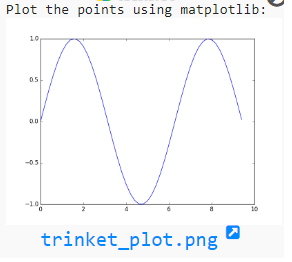﻿ NumPy: Compute the x and y coordinates for points on a sine curve and plot the points using matplotlib - w3resource

# NumPy: Compute the x and y coordinates for points on a sine curve and plot the points using matplotlib

## NumPy: Basic Exercise-40 with Solution

Write a NumPy program to compute the x and y coordinates for points on a sine curve and plot the points using matplotlib.

Sample Solution :

Python Code :

``````import numpy as np
import matplotlib.pyplot as plt
# Compute the x and y coordinates for points on a sine curve
x = np.arange(0, 3 * np.pi, 0.2)
y = np.sin(x)
print("Plot the points using matplotlib:")
plt.plot(x, y)
plt.show()
``````

Sample Output:

``````

Python Code Editor:

Have another way to solve this solution? Contribute your code (and comments) through Disqus.

What is the difficulty level of this exercise?

﻿

Inviting useful, relevant, well-written and unique guest posts